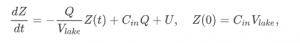# 1.7 Calculation

Course subject(s) 1. Introducing Mathematical Modelling

In the previous section, you have derived a mathematical model for Z(t), the mass of the pollutant in the lake:where QVlakeCin and U all have constant values.

In this section, you will not solve the differential equation analytically (which is not that difficult, but it is beyond the scope of this course). You will also not approximate the solution numerically (as you will learn to do in the next section). In this section, you are going to investigate the solution just by using the phase line.